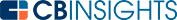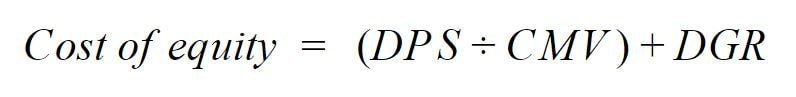# What is the Cost of Equity Formula?The cost of equity formula helps investors and companies calculate rates of return. From an investor’s perspective, it calculates the rate of return they require from their investment in a specific equity. From a company’s standpoint, it calculates the required return from a specific investment or project.

When companies need to raise money, their two primary tools are equity and debt. Equity is a more expensive option; however, it usually offers a much better rate of return. You can calculate the cost of equity with dividends or assets.

## Calculating the cost of equity with dividends

Calculating the cost of equity is much simpler when a company pays dividends. The calculation is based on the expected future dividends the company has committed to paying. This formula only interprets those dividend obligations as costs, however, which can be limiting.

For the dividend cost of equity calculation, use the following variables:

• DPS (dividend per share)
• CMV (current market value)
• DGR (dividend growth rate)For a fictitious example, let’s say XYZ Corp has an expected dividend next year of \$1 a share, its current market value is \$10, and the dividend historically grows at 1%. The calculation would look like this:

(\$1 / \$10) + 1% = 10% cost of equity

## Figuring the cost of equity with assets

The capital asset pricing model (CAPM) formula is more complicated, but it can be used for companies that do not pay dividends. This model uses historical data instead of future data and may be considered less exact. This formula strives to determine whether an investment is valued fairly based on the amount of time and risk it has against the expected return.

The CAPM compares the cash flow of a company to others of a similar size and with similar characteristics. Since this formula factors in competitors, companies may use it to determine the minimum level at which they must maintain their stock price to retain investors.

The CAPM is made up of three kinds of returns:

The risk-free return rate is normally based on US government securities such as treasury bonds. An average return can be from any broad group such as an ETF, the SPY, or the Dow.

The differential return is a calculation based on the company’s volatility against the broader market. This number is published by various financial services as +/- 1. A beta value less than 1.0 shows the company has less risk, while a beta value greater than 1.0 shows it is riskier than the market average.

With that said, we’ll use the following variables:

• RFR (risk-free return)
• B (beta)
• AVG (average stock return)

Thus, the CAPM cost of equity formula is:

Cost of equity = RFR + (B x (AVG - RFR))

Let’s use the fictitious company of ZYX Corp with a 1.2 beta. The risk-free rate is 3%, and the return on the S&P500 is 9%. The calculation is:

3% + (1.2 x (9% - 3%)) = 10.2%

The cost of equity formula is useful for both investors and companies to evaluate the expected return on an investment. Companies use this calculation to determine whether a project or acquisition will benefit the company and shareholders in time, as well. When companies pay dividends, the cost of equity formula is based on the future dividend payments expected. Without dividends, the formula uses historical rates of return and risks compared to the broader market.# www.cbinsights.com